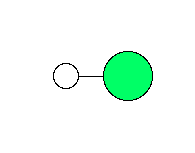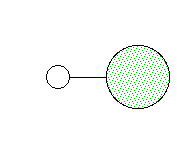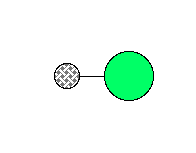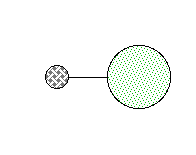## H-D fractionation between HF and HCl

A simple example of how vibrational frequencies can be used to estimate equilibrium isotope fractioantion factors is hydrogen-deuterium (1H-2H) substitution in HCl and HF.  The vibrational frequencies and zero-point energies of both hydrogen- and deuterium-bearing molecules are shown below.  Note that the heavy isotope of hydrogen, 2H, is often called deuterium or simply "D":

### o = 2991 cm-1 ZPE = ho/2 = 17898 J/mol### o = 2145 cm-1 ZPE = ho/2 = 12836 J/molThe hydrogen-deuterium exchange reaction looks like this:

2HCl + 1HF <-> 1HCl + 2HF
Because the electronic structures of the two hydrogen isotopes are essentially identical, the driving energy of this reaction is simply the sum of the vibrational energies of the products minus the vibrational energies of the reactants.  If we assume that all molecules are in the lowest-energy vibrational state, the vibrational energy is equal to the zero-point energy.  So:
DGrxn = (17898 J/mol + 17940 J/mol) - (12836 J/mol + 24768 J/mol)
= -1766 J/mol
This means that the reaction will go towards the right, concentrating deuterium in HF.  This is expected, because the HF vibrates at a much higher frequency than HCl (or just about anything else, for that matter).
Of course, at temperatures above absolute zero it is necessary to take account of excited vibrational states, using the vibrational partition function.  Molecular rotations and translations also play a role in equilibrium isotope fractionation, but rotational and translational quanta are smaller than vibrational quanta, and are generally much less important.